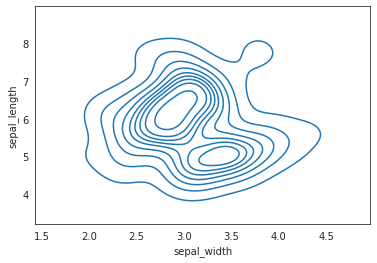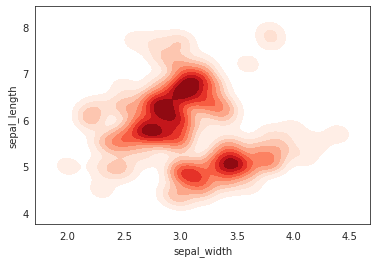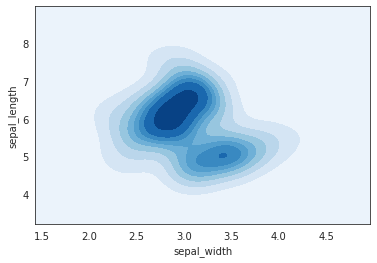# Contour Plot using Seaborn

This post explains how to draw a contour plot(density plot) using kdeplot() function of seaborn library.

The following code produces 3 contour plots using seaborn python library. You have to provide 2 numerical variables as input (one for each axis). The function will calculate the kernel density estimate and represent it as a contour plot or density plot. The aguments of the function `kdeplot()` are:

• `x, y` : Variables that specify positions on the x and y axes.
• `shade` : Controls the presence of a shade.
• `cmap` : Colormap.
• `bw_adjust` : Bandwidth, smoothing parameter.
• `thresh` : number in [0, 1], Lowest iso-proportion level at which to draw a contour line.
``````# libraries & dataset
import seaborn as sns
import matplotlib.pyplot as plt

# set seaborn style
sns.set_style("white")

# Basic 2D density plot
sns.kdeplot(x=df.sepal_width, y=df.sepal_length)
plt.show()👋 This document is a work by Yan Holtz. Any feedback is highly encouraged. You can fill an issue on Github, drop me a message onTwitter, or send an email pasting `yan.holtz.data` with `gmail.com`.